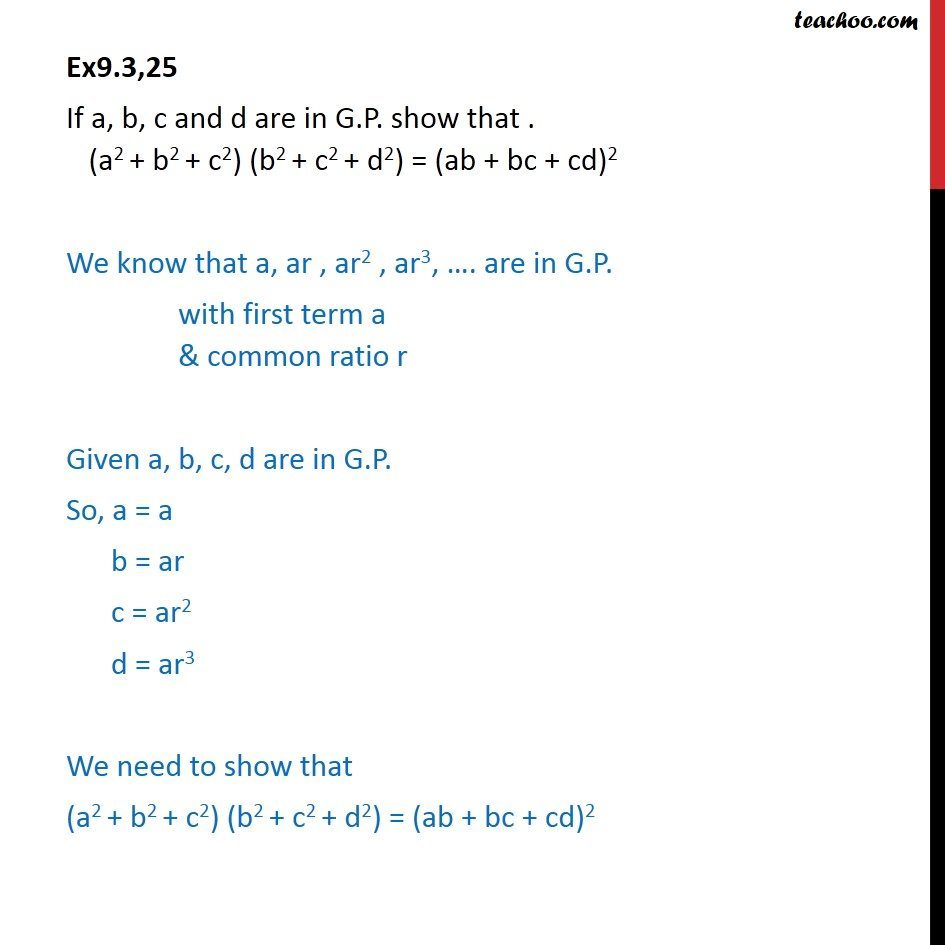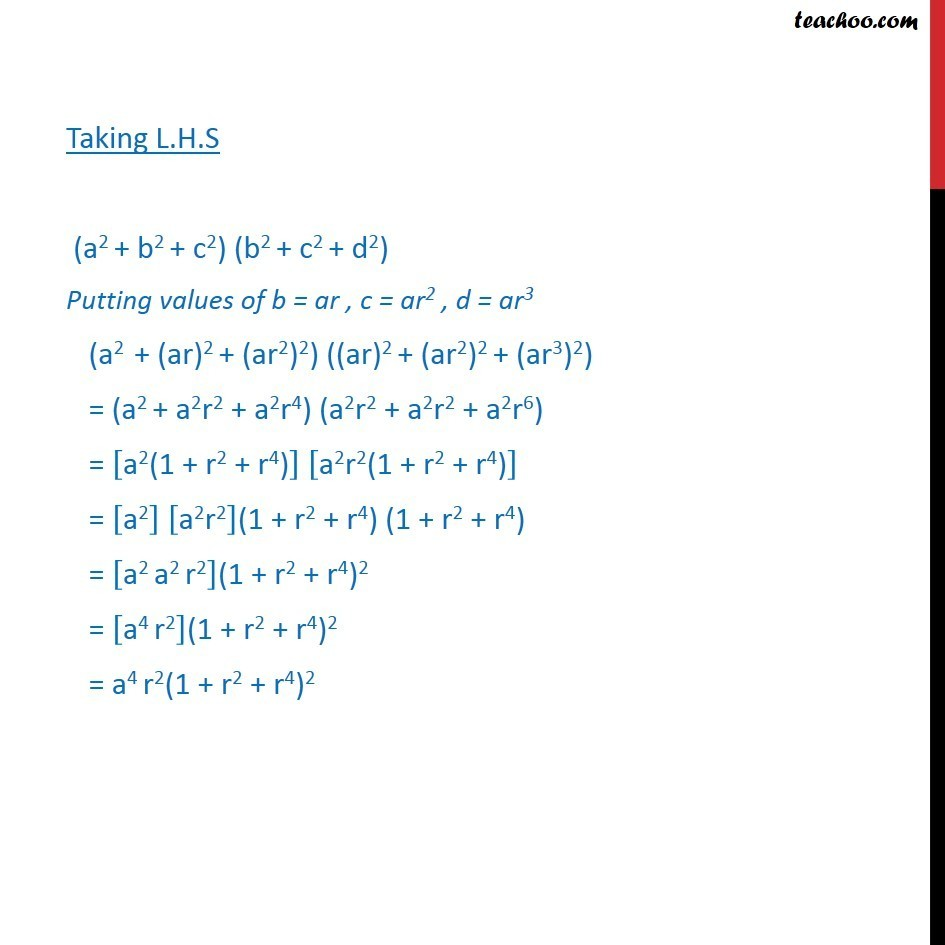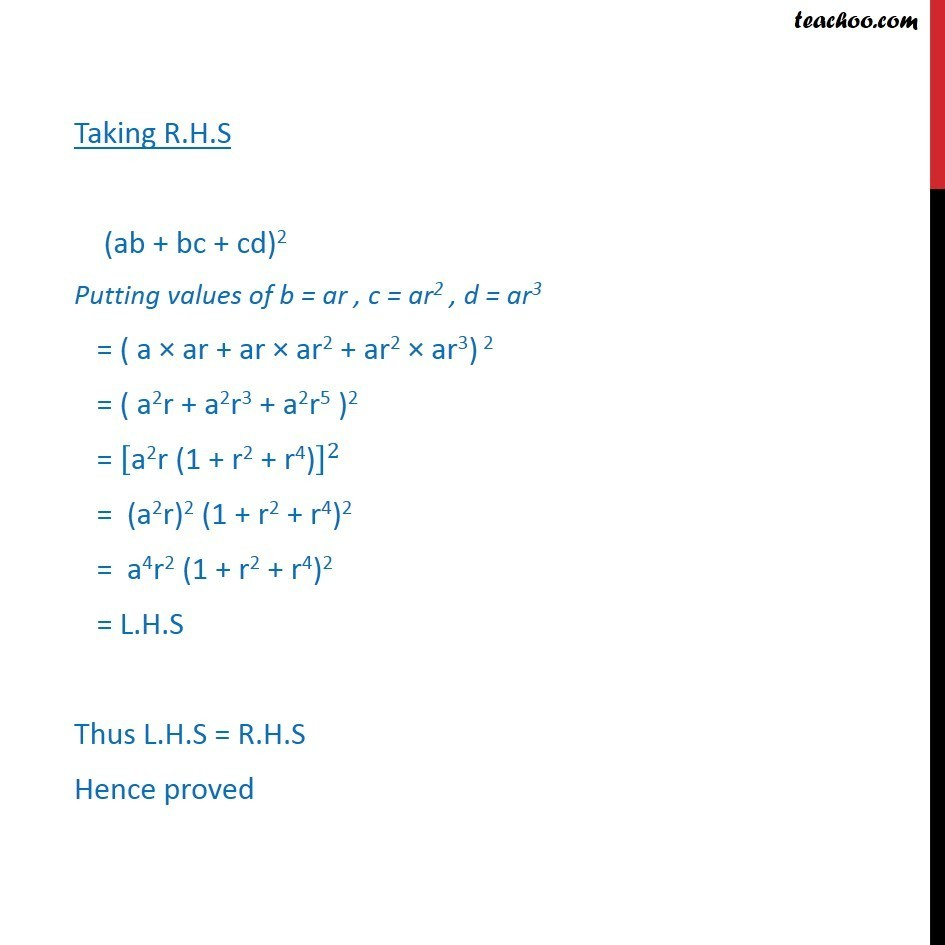Ex 8.2

Chapter 8 Class 11 Sequences and Series
Serial order wiseLearn in your speed, with individual attention - Teachoo Maths 1-on-1 Class

### Transcript

Ex9.3,25 If a, b, c and d are in G.P. show that . (a2 + b2 + c2) (b2 + c2 + d2) = (ab + bc + cd)2 We know that a, ar , ar2 , ar3, …. are in G.P. with first term a & common ratio r Given a, b, c, d are in G.P. So, a = a b = ar c = ar2 d = ar3 We need to show that (a2 + b2 + c2) (b2 + c2 + d2) = (ab + bc + cd)2 Taking L.H.S (a2 + b2 + c2) (b2 + c2 + d2) Putting values of b = ar , c = ar2 , d = ar3 (a2 + (ar)2 + (ar2)2) ((ar)2 + (ar2)2 + (ar3)2) = (a2 + a2r2 + a2r4) (a2r2 + a2r2 + a2r6) = ["a2(1 + r2 + r4)" ] ["a2r2(1 + r2 + r4)" ] = ["a2" ] ["a2r2" ]"(1 + r2 + r4)" "(1 + r2 + r4)" = ["a2 a2 r2" ]"(1 + r2 + r4)"2 = ["a4 r2" ]"(1 + r2 + r4)"2 = "a4 r2(1 + r2 + r4)"2 Taking R.H.S (ab + bc + cd)2 Putting values of b = ar , c = ar2 , d = ar3 = ( a × ar + ar × ar2 + ar2 × ar3) 2 = ( a2r + a2r3 + a2r5 )2 = ["a2r (1 + r2 + r4)" ]^2 = (a2r)2 (1 + r2 + r4)2 = a4r2 (1 + r2 + r4)2 = L.H.S Thus L.H.S = R.H.S Hence proved